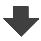Search:

# The formation of rust

Why does water rise in the tube?

Because part of the air has been consumed: Oxygen.

Rust is iron(III)oxide trihydrate. Formula?

Rust = Iron(III)oxide trihydrate = $Fe_2O_3\cdot3H_2O$.

Write down the equation of this reaction!

$4Fe$ $+$ $3O_2$ $+$ $6H_2O$ $\longrightarrow$ $2Fe_2O_3\cdot3H_2O$.

What is this type of reaction?

It's an oxidation.